# PivotGridFieldBase.SummaryType Property

Gets or sets the type of the summary function which is calculated against the current data field.

Namespace: DevExpress.XtraPivotGrid

Assembly: DevExpress.PivotGrid.v23.1.Core.dll

NuGet Packages: DevExpress.PivotGrid.Core, DevExpress.Win.Dashboard.Design

## Declaration

``````[DefaultValue(PivotSummaryType.Sum)]
public PivotSummaryType SummaryType { get; set; }``````

## Property Value

Type Default Description
PivotSummaryType Sum

A PivotSummaryType enumeration value that specifies the summary function type which is calculated against the current field.

Available values:

Show 13 items
Name Description
Count

The number of values (excluding Null and DBNull values).

Sum

The sum of the values.Min

The smallest value.

Max

The largest value.

Average

The average of the values.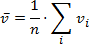StdDev

An estimate of the standard deviation of a population, where the sample is a subset of the entire population.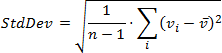StdDevp

The standard deviation of a population, where the population is all of the data to be summarized.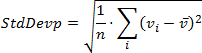Var

An estimate of the variance of a population, where the sample is a subset of the entire population.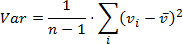Varp

The variance of a population, where the population is all of the data to be summarized.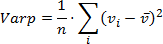Custom

Specifies whether calculations should be performed manually, by handling the CustomSummary event.

Not supported in OLAP and server mode.

CountDistinct

The number of distinct values. This function works only in the Optimized mode.

Median

The median of the values. A median is the number separating the higher half of a value range from the lower half. This function works only in the Optimized mode.

Mode

The mode of the values. A mode is the value that is repeated more often than any other. This function works only in the Optimized mode.

## Remarks

Note

Custom summary type is not supported in Optimized and Server modes. Use ExpressionDataBinding instead.

In PivotGridControl, each cell can contain summarized data from several rows in a corresponding data source. PivotGridControl uses summary calculation methods like Sum, Average, Count, Min, Max, and so on against such data fields. The field’s `SummaryType` property determines the summary function type.

The String and DateTime types do not support arithmetic addition. For fields that do not contain numeric data only the following summary functions can be calculated: `Count`, `Min`, and `Max`. If the field’s `SummaryType` property is set to any other value, the corresponding data cells displays `0`.

The numeric data fields support all available summary function types.

Note

The SummaryType property is not in effect in the OLAP mode. The summary type of a measure is controlled by Analysis Services itself; it is not possible to customize it in the PivotGrid control.

The following code snippets (auto-collected from DevExpress Examples) contain references to the SummaryType property.

Note

The algorithm used to collect these code examples remains a work in progress. Accordingly, the links and snippets below may produce inaccurate results. If you encounter an issue with code examples below, please use the feedback form on this page to report the issue.